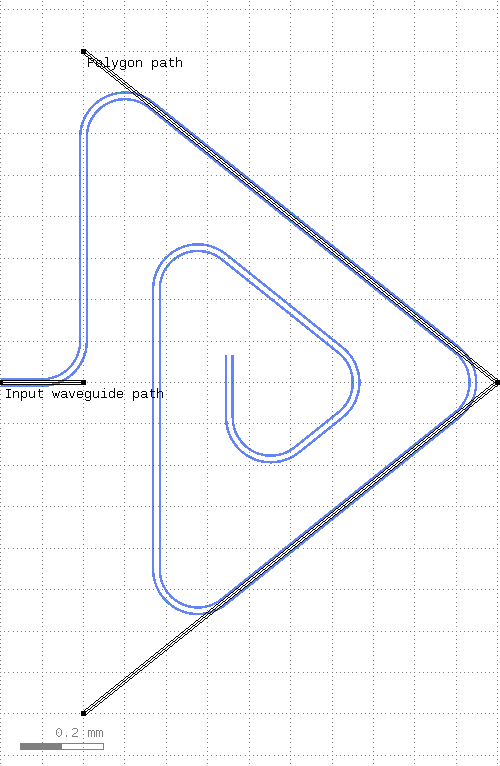# kqcircuits.elements.spiral_resonator_polygon¶

class kqcircuits.elements.spiral_resonator_polygon.SpiralResonatorPolygon[source]

The PCell declaration for a polygon shaped spiral resonator.

The resonator waveguide starts at the first point of self.input_path and goes through each each of its points. The last point of self.input_path will connect to the first point of self.poly_path, unless the length of self.input_path is already longer than self.length.

The polygon shape is defined by self.poly_path, where each point is a vertex of the polygon. The points should either be in clockwise or counter-clockwise order. The waveguide will first continue along the polygon edges, and then spiral inside the polygon such that each segment will be parallel to one of the edges defined by self.polygon_path.

Note, that if you want the segment from self.input_path to self.poly_path to be “a part of the spiral” (following the polygon shape), you will need to choose the points such that the edge self.input_path[-1] - self.poly_path is parallel to the edge self.poly_path - self.poly_path[-1].

The spacing between waveguides in the spiral can either be chosen manually or automatically. The automatic spacing attempts to find the largest possible spacing.

Airbridges can optionally be placed with a given spacing along the resonator waveguide by setting non-zero value to bridge_spacing. Alternatively, one can independently set number of airbridges on each straight segment of spiral polygon by using parameter n_bridges_pattern.

Non-negative value to connector_dist inserts face-to-face connector to spiral resonator so that the beginning and the end of resonator will be on different faces.

build()[source]

Child classes re-define this method to build the PCell.

kqcircuits.elements.spiral_resonator_polygon.rectangular_parameters(above_space=500, below_space=400, right_space=1000, x_spacing=100, y_spacing=100, bridges_left=False, bridges_bottom=False, bridges_right=False, bridges_top=False, r=100, **kwargs)[source]

A utility function to easily produce rectangular spiral resonator (old SpiralResonatorRectangle).

Parameters
• above_space – Space above the input (µm)

• below_space – Space below the input (µm)

• right_space – Space right of the input (µm)

• x_spacing – Spacing between vertical segments (µm)

• y_spacing – Spacing between horizontal segments (µm)

• bridges_left – Crossing airbridges left

• bridges_bottom – Crossing airbridges bottom

• bridges_right – Crossing airbridges right

• bridges_top – Crossing airbridges top

• r – Turn radius (µm)

Returns

dictionary of parameters for SpiralResonatorPolygon

PCell parameters:

• length (Double) - Resonator length, default=`5000`, unit=`μm`

• input_path (Shape) - Input waveguide path, default=`(-200,0;0,0) w=10 bx=0 ex=0 r=false`

• poly_path (Shape) - Polygon path, default=`(0,800;1000,0;0,-800) w=10 bx=0 ex=0 r=false`

• auto_spacing (Boolean) - Use automatic spacing, default=`True`

• manual_spacing (List) - Manual spacing pattern, default=``, unit=`[μm]`

• bridge_spacing (Double) - Airbridge spacing, default=`0`, unit=`μm`

• n_bridges_pattern (List) - Pattern for number of airbridges on edges, default=``

• connector_dist (Double) - Negative value means single face resonator without connector., default=`-1`, unit=`µm`

• term1 (Double) - Termination length start, default=`0`, unit=`μm`

• term2 (Double) - Termination length end, default=`0`, unit=`μm`

• a (Double) - Width of center conductor, default=`10`, unit=`μm`

• b (Double) - Width of gap, default=`6`, unit=`μm`

• n (Int) - Number of points on turns, default=`64`

• r (Double) - Turn radius, default=`100`, unit=`μm`

• margin (Double) - Margin of the protection layer, default=`5`, unit=`μm`

• face_ids (List) - Chip face IDs list, default=`['1t1', '2b1', '1b1', '2t1']`

• display_name (String) - Name displayed in GUI (empty for default), default=

• protect_opposite_face (Boolean) - Add opposite face protection too, default=`False`

• airbridge_type (String) - Airbridge type, default=`Airbridge Rectangular`, choices=`['Airbridge Rectangular', 'Airbridge Multi Face']`

• bridge_width (Double) - Bridge width, default=`40`, unit=`μm`

• pad_length (Double) - Pad length, default=`40`, unit=`μm`

• bridge_length (Double) - Bridge length (from pad to pad), default=`44`, unit=`μm`

• include_bumps (Boolean) - Include bumps, default=`True`

• ubm_diameter (Double) - Under-bump metalization diameter, default=`40`, unit=`μm`

• bump_diameter (Double) - Bump diameter, default=`25`, unit=`μm`